21.1
20.2
20.1
19.2
19.1
18.2
18.1
17.2
.NET Framework 4.5.2+
.NET Framework 4.5.2+
.NET Standard 2.0+
A newer version of this page is available. Switch to the current version.

# PivotDataConsolidationFunction Enum

Lists aggregation functions to apply to data fields in a PivotTable report.

Namespace: DevExpress.Spreadsheet

Assembly: DevExpress.Spreadsheet.v19.1.Core.dll

## Declaration

``public enum PivotDataConsolidationFunction``

## Members

Name Description
Average

The average of the values.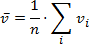Count

The number of nonempty values.

CountNumbers

The number of numeric values.

Max

The largest value.

Min

The smallest value.

Product

The product of the values.

StdDev

An estimate of the standard deviation of a population, where the sample is a subset of the entire population.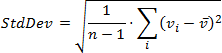StdDevp

The standard deviation of a population, where the population is all of the data to be summarized.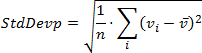Sum

The sum of the values.

Var

An estimate of the variance of a population, where the sample is a subset of the entire population.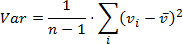Varp

The variance of a population, where the population is all of the data to be summarized.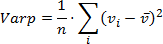## Remarks

The PivotDataConsolidationFunction enumeration members are used by the PivotDataField.SummarizeValuesBy property.

See Also